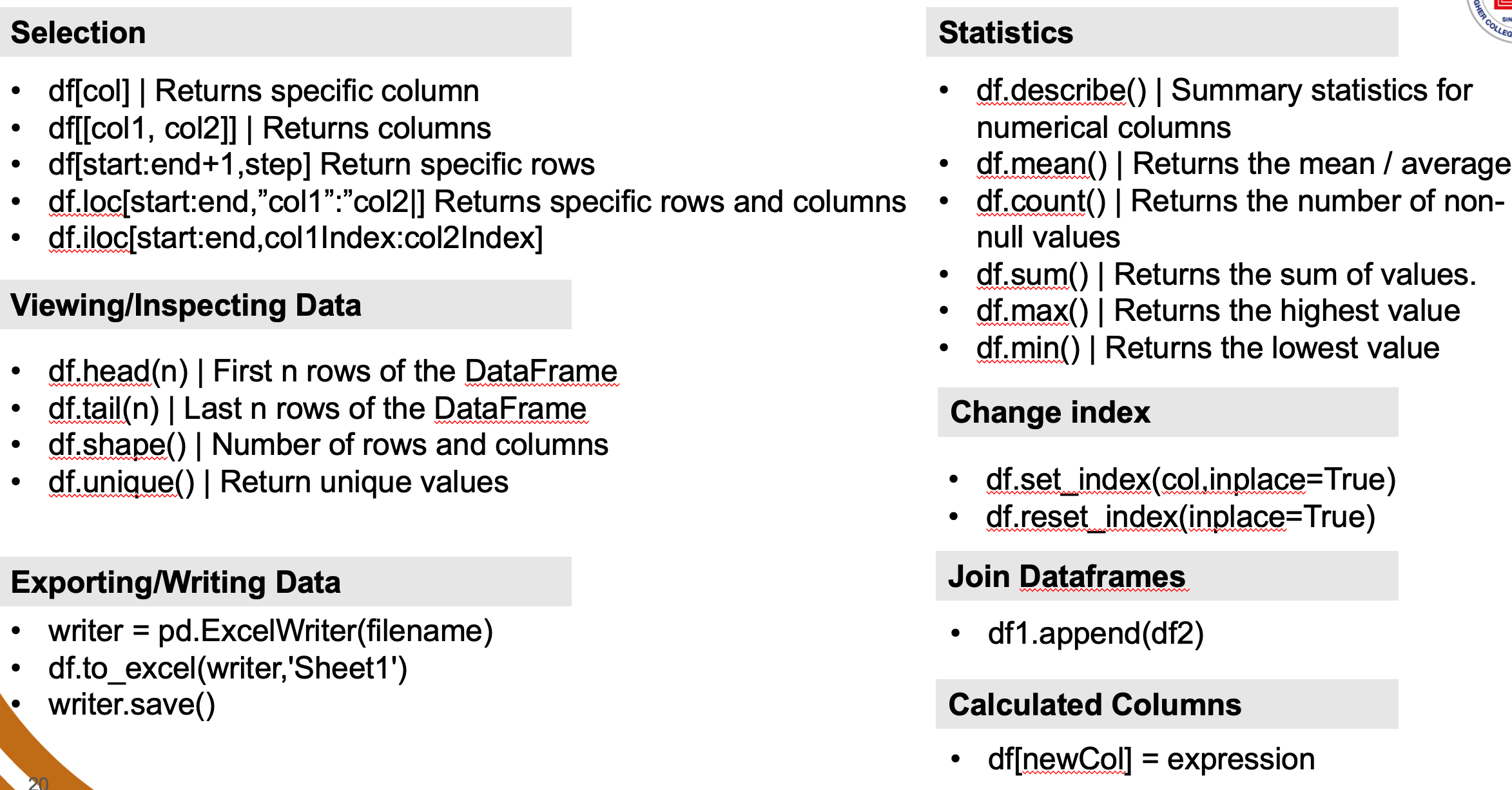# Data Analytics Part-2

## Exploring the data: Show information about the data frame

### Example: Show information about the data frame

#### Display all the rows and columns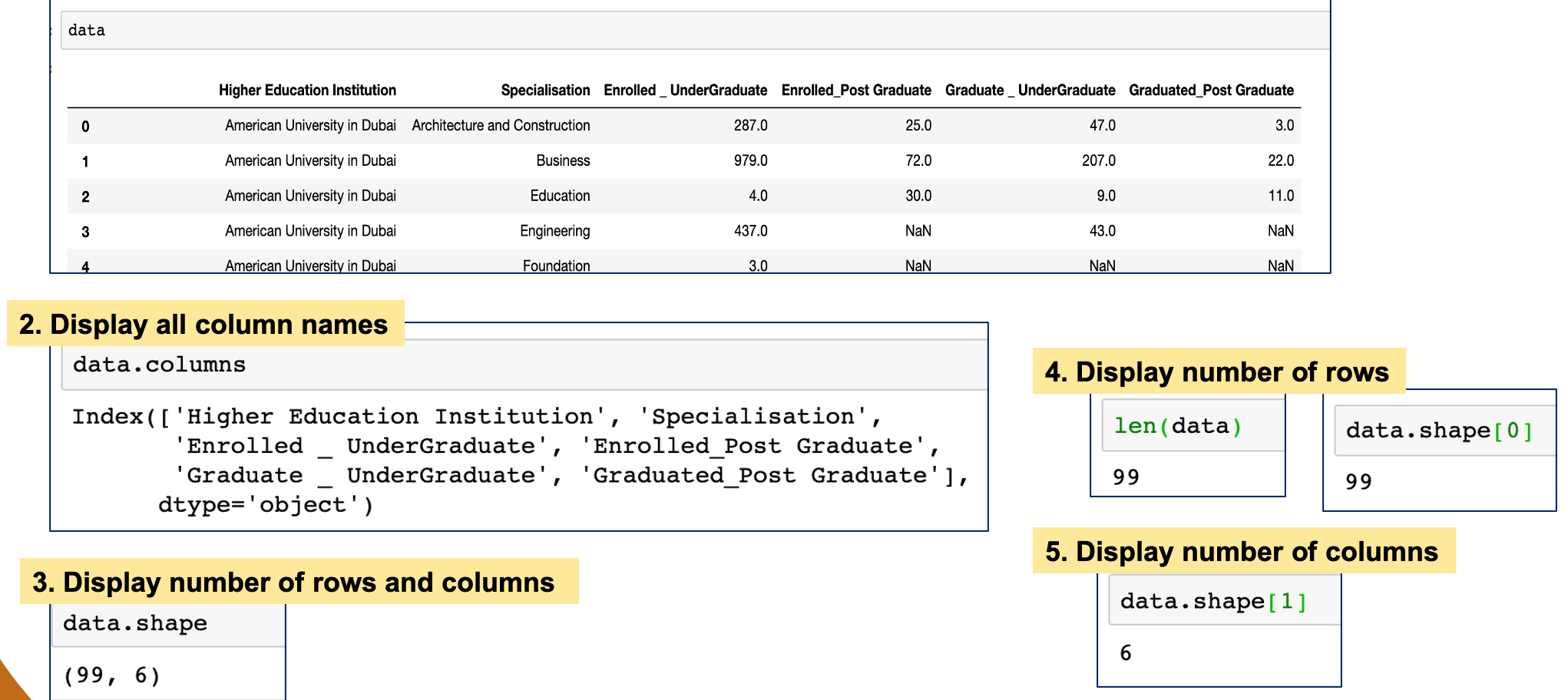## Slicing the data: Show specific rows / columns of a data frame

Slicing data is a technique which is used to create small sets of your large data.

• dataFrame.head(): Display first 5 rows of the data frame.
• dataFrame.head(n): Display first n rows of the data frame. [ n is an int value]
• dataFrame.tail(): Display last 5 rows of the data frame.
• dataFrame.tail(n): Display last n rows of the data frame. [ n is an int value]
• dataFrame[start:end+1]: Display all rows start from index start to index end
• dataFrame[start:end+1:step]: Display all rows start from index start to index end in the intervals of step
• dataFrame[" Column "]: Display specific column
• dataFrame [[" Column1 ", " Column2 ",…]]: : Display specific columns

### Example: Show specific rows of the data frame.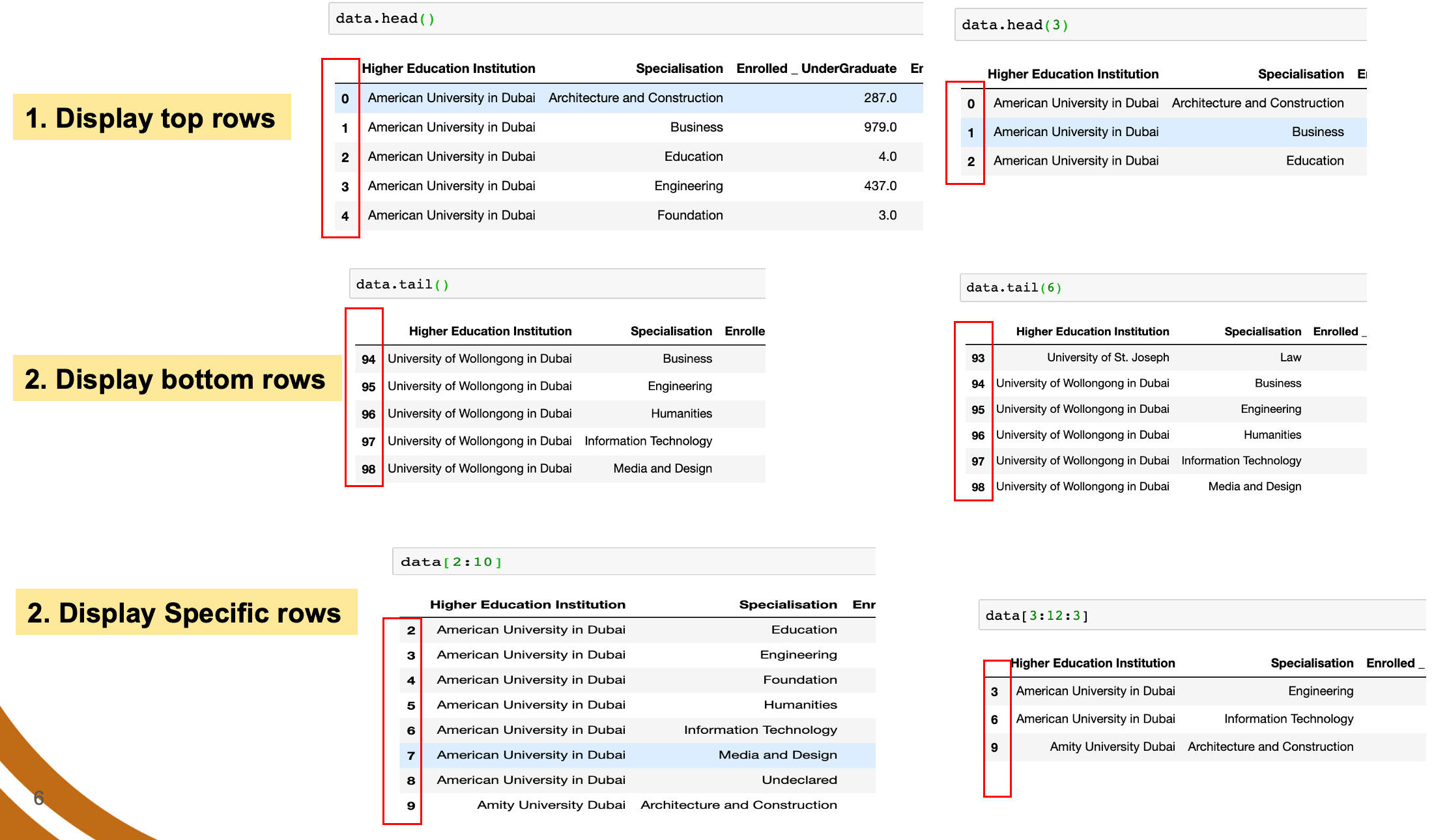### Example: Show specific columns of the data frame.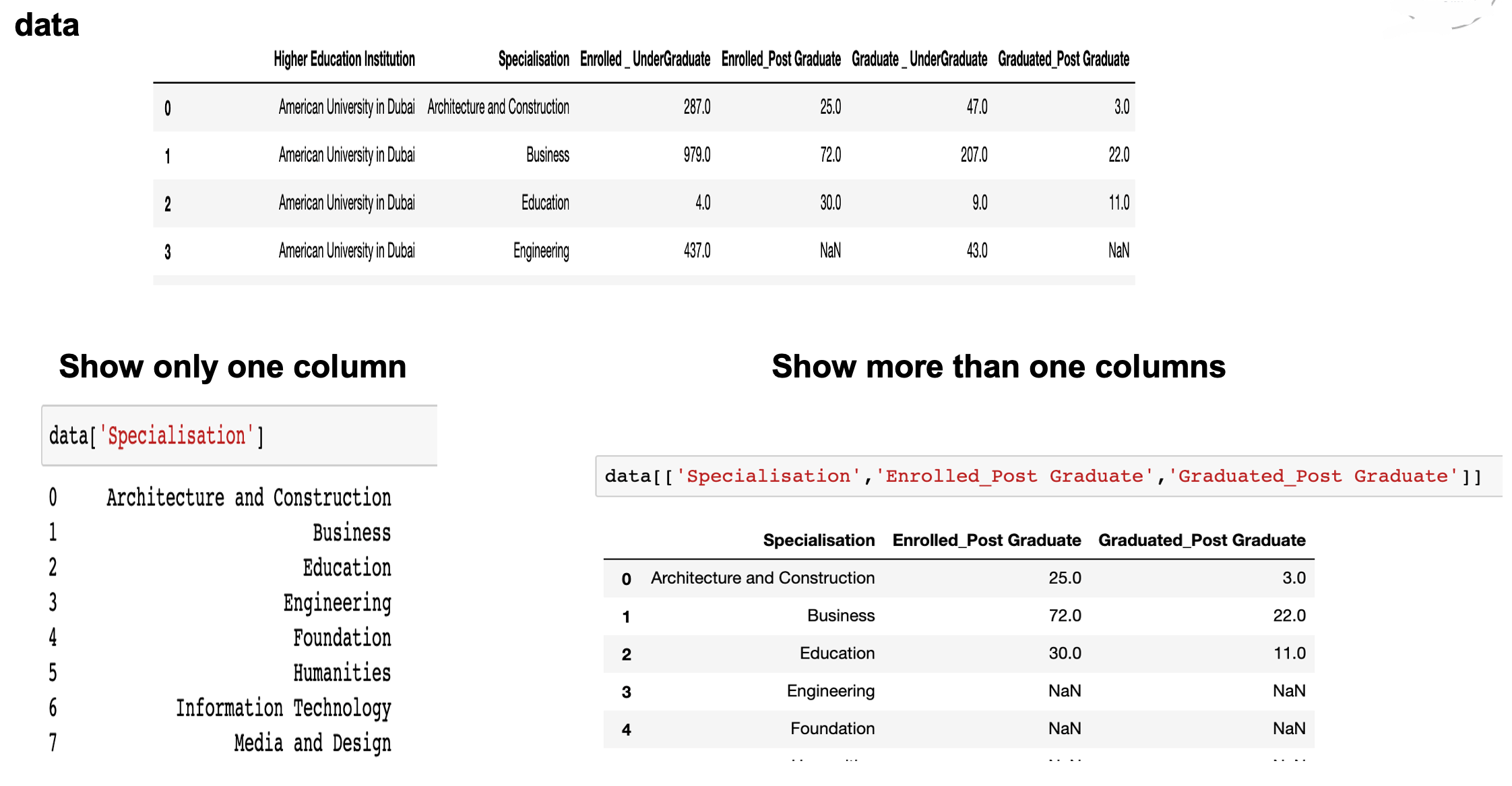## Slice data using loc

The pandas loc function allows us to search and slice data based on both index and columns. It is a powerful tool to allow us to focus on the important rows and columns for our data analytics.

```	    dataframe.loc[starting_row:end_row,starting_column:end_column]
```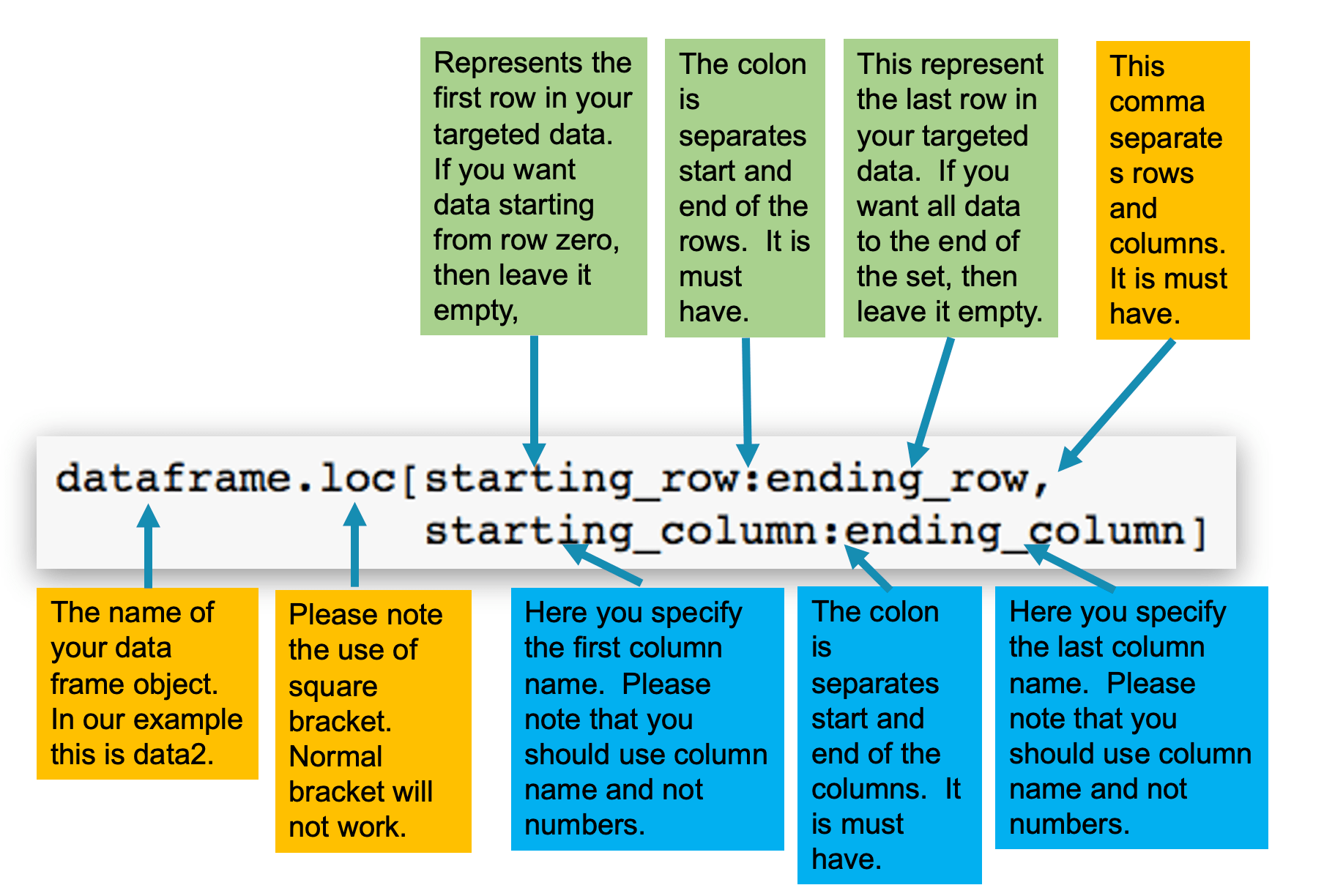### Example: Slice data using loc

The following code will display rows 2 to 5 and columns "Higher Education Institution" to "Enrolled_Post Graduate"

```	    data.loc[2:5,"Higher Education Institution": "Enrolled_Post Graduate"]
```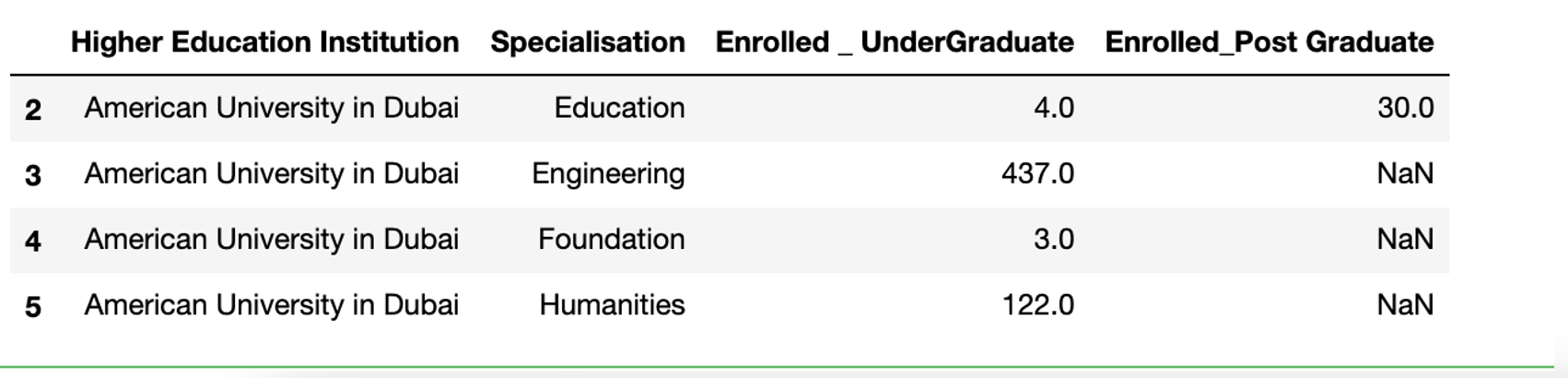• Note that you need to use the index of the rows and the name of the column.
• In this example the index is 2:5
• The column "Higher Education Institution" :"Enrolled_Post Graduate"

## Slice data using loc

You can display columns that are not in sequence, you need to add then inside a square bracket [ ].

### Example: Display rows 3, 5, and 5 and Columns "Higher Education Institution" and "Enrolled _ UnderGraduate"

```	    data.loc[[3,5,7],["Higher Education Institution", "Enrolled _Under Graduate"]
```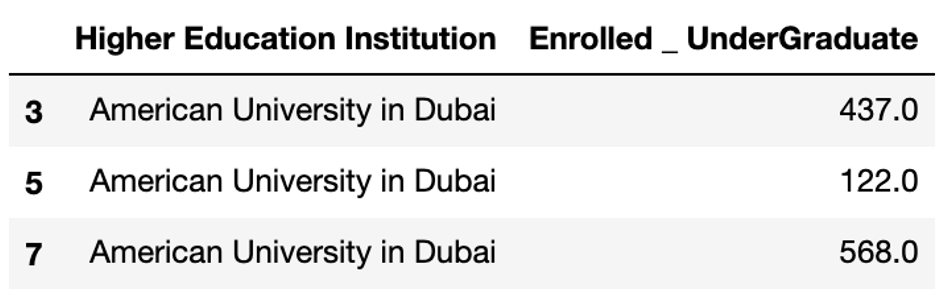## Slice data using iloc

The pandas iloc function similar to loc to slice rows and columns, it use index for columns instead of column names.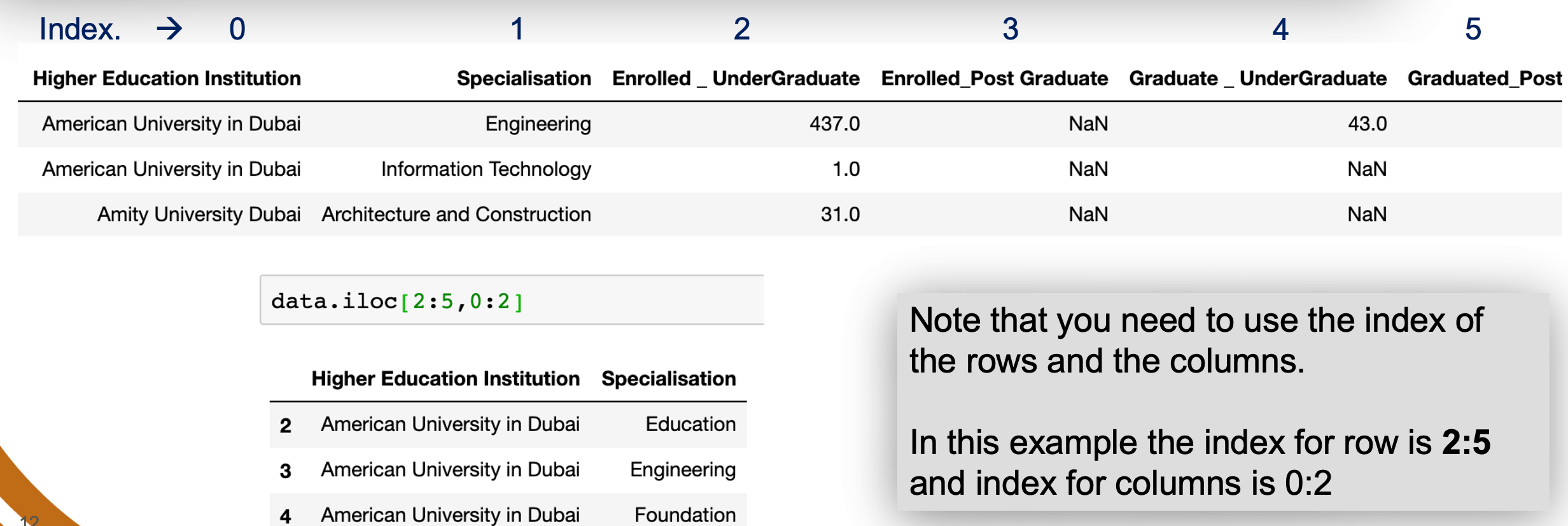## Changing index

The default index in a DataFrame is integer values starting from zero. To change the default index to any other column, you need to use .set_index as follows:

```	    data.set_index("igher Education Institution",inplace=True)
```

### Example: Change the the index of our test example to StudentID

```	    data.set_index("ColumnName",inplace=True)
```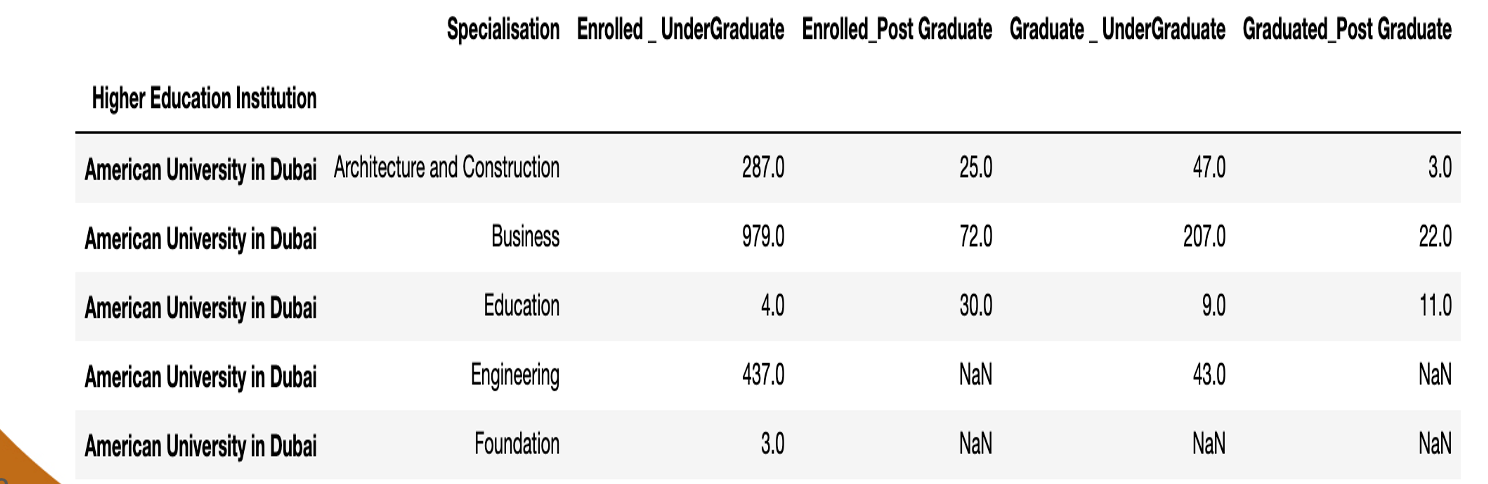Note: Higher Education Institution is now the index and presented different on the DataFrame. The column is appearing in bold.

### Example: Resetting the index

When you need to reset the index back to its original values. There are different ways to do this. On common method is to run the line that reads the data from your source. However, you can use the function: .reset_index()

Reset the index of our test example to its original values

```	    data.reset_index(inplace=True)
```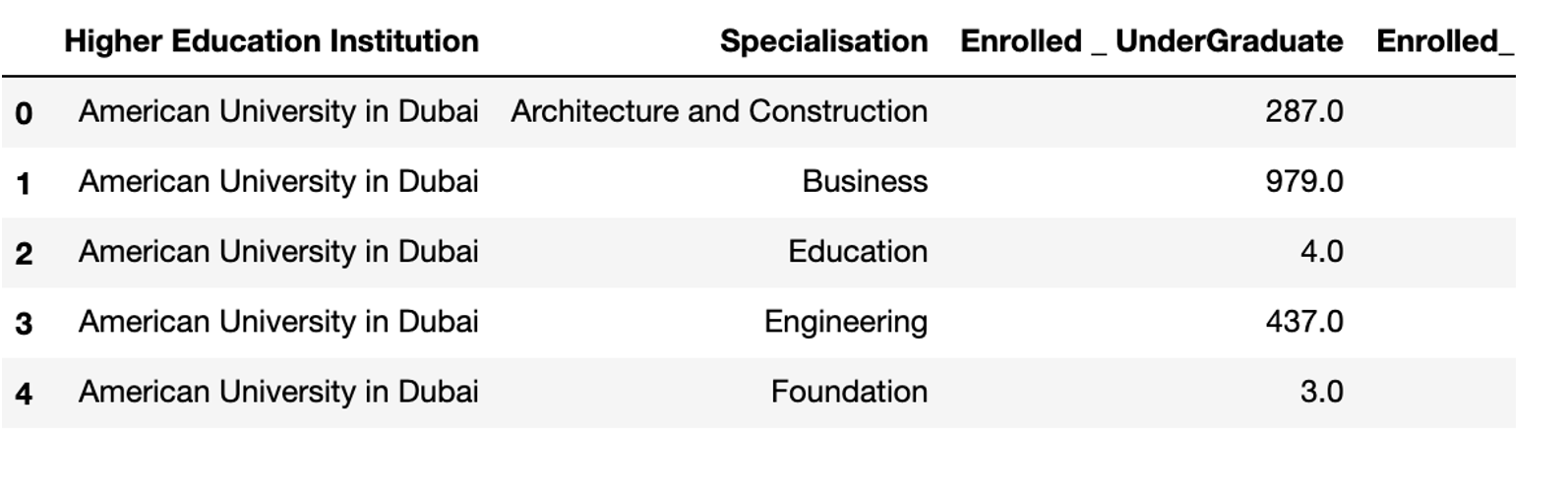## Statistics / Aggregation Commands

When you need to summaries the data in data frame Pandas makes the calculation of different statistics very simple.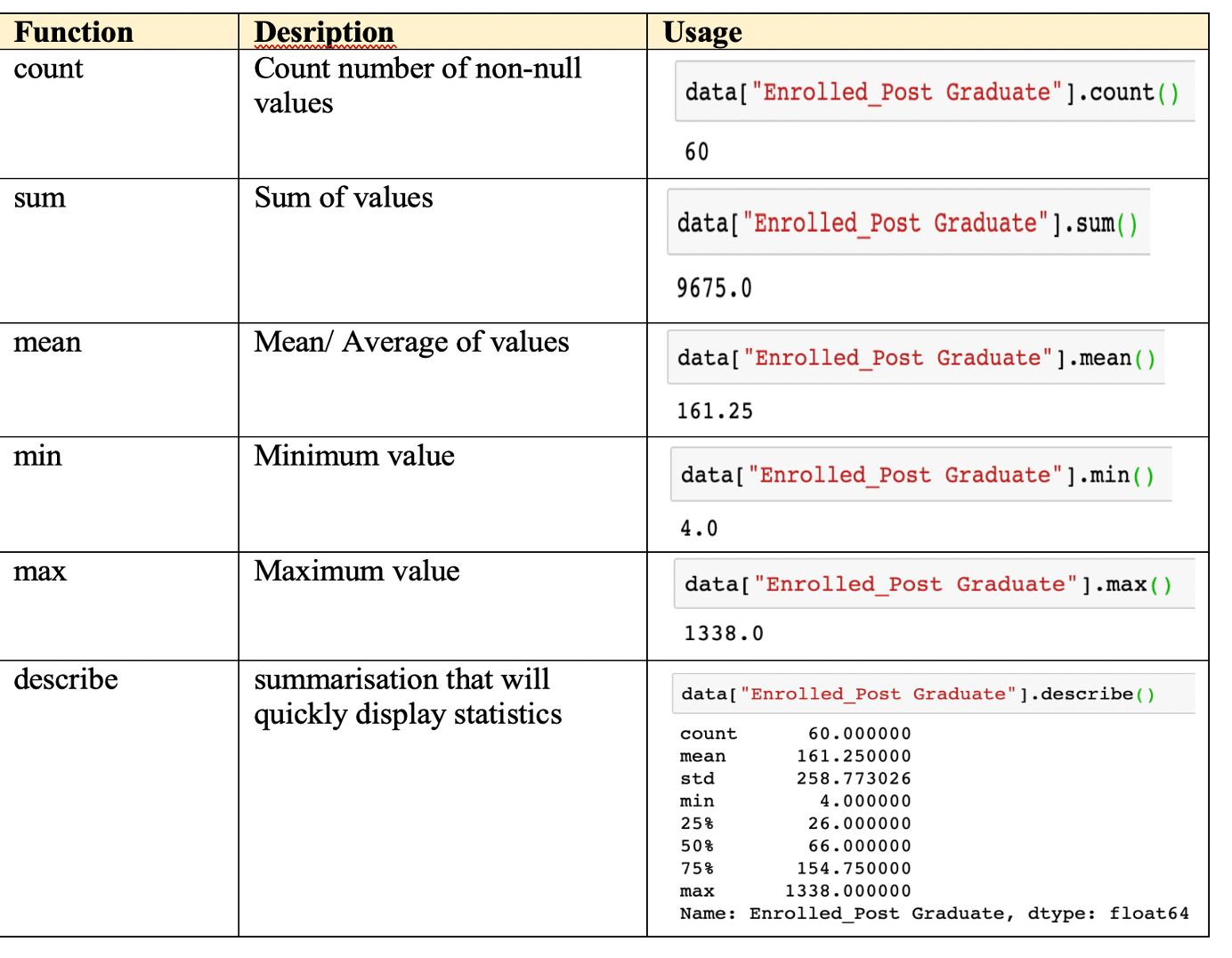### Syntax of using statistic command on specific column

```	    dataframe["Column"].statistics_method()
```

### Syntax of using statistic command on all column

```	    dataframe.statistics_method()
```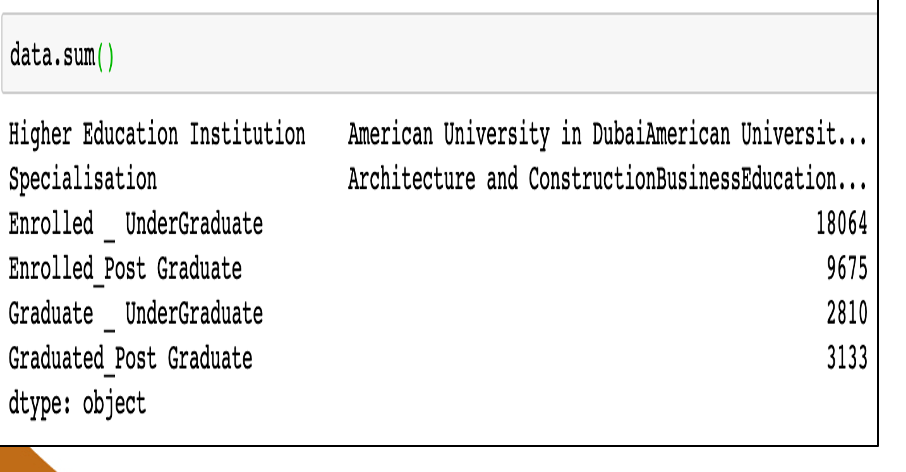## Displaying unique values in a column

Finding unique (nonrepeating) values in a column is needed to perform analysis on your data.

```	    dataFrame["Column"].unique
```

For example, to know the unique values in the column "Specialisation" use the function .unique() that helps you with perform this task.

```	    data["Specialisation"].unique
```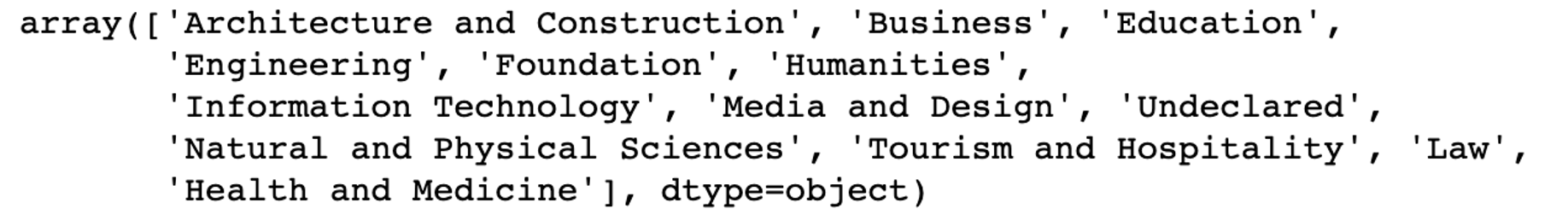## Calculated Columns

Pandas allows you to easily add new columns to the DataFrame. This is usually used to create a new calculated column.

Syntax to create a new column:

```	    DataFrame["New Column"] = expression
```

### Example: The below example create a new column Total Enrolled which is sum of Enrolled Graduates and Enrolled Post Graduate

```	    data[" Total Enrolled"] = data[" Enrolled _ Undergraduate"] + data[" Enrolled_Post Greauate"]
```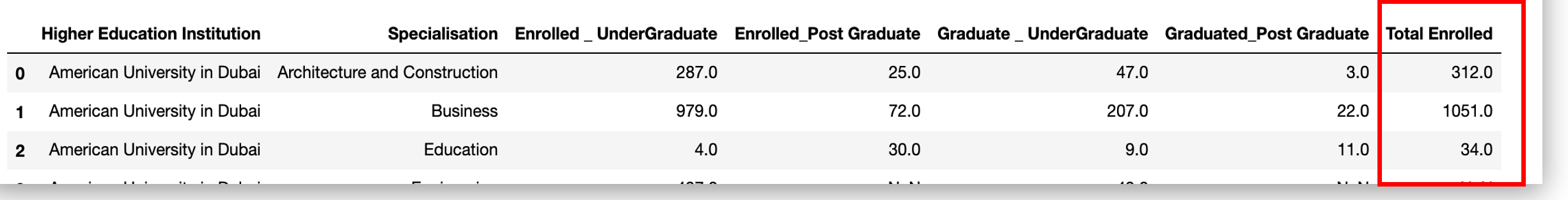## Appending Data : Join two Dataframe

```	    newDataFrame = dataFrame1.append(dataFrame2)
```

### Example: You have two data frames as bellow. Both sheet have the same structure. They contains students from Education and Foundation Specialisation. We need to combine both in one DataFrame.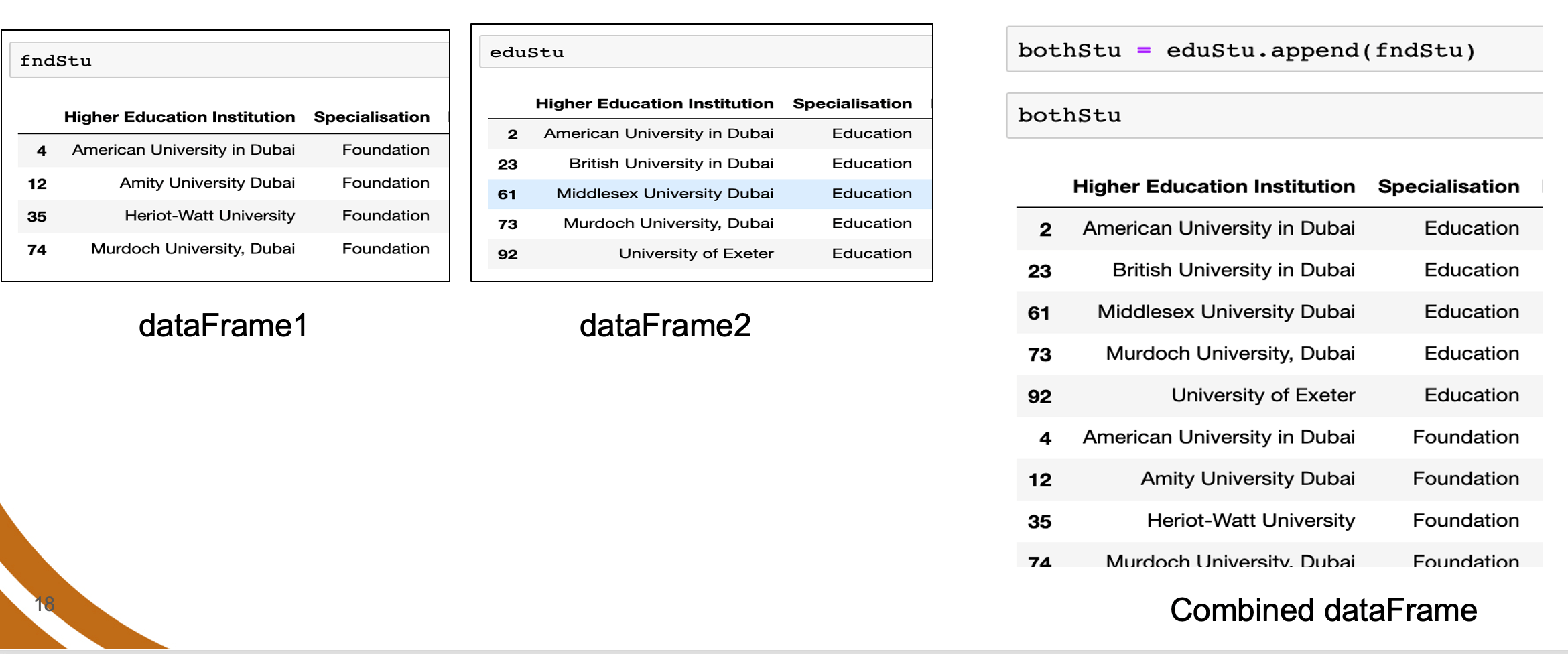## Writing data to external file

### Example: Write the data you cleaned in the previous example to an external file.

```	    writer = pd.ExcelWriter(' NewData.xlsx ')
data.to_excel(Writer,'sheet1 ')
Writer.save()
```

The above lines stores the DataFrame data in the an excel file 'NewData.xlsx' in a sheet with the name 'Sheet1'.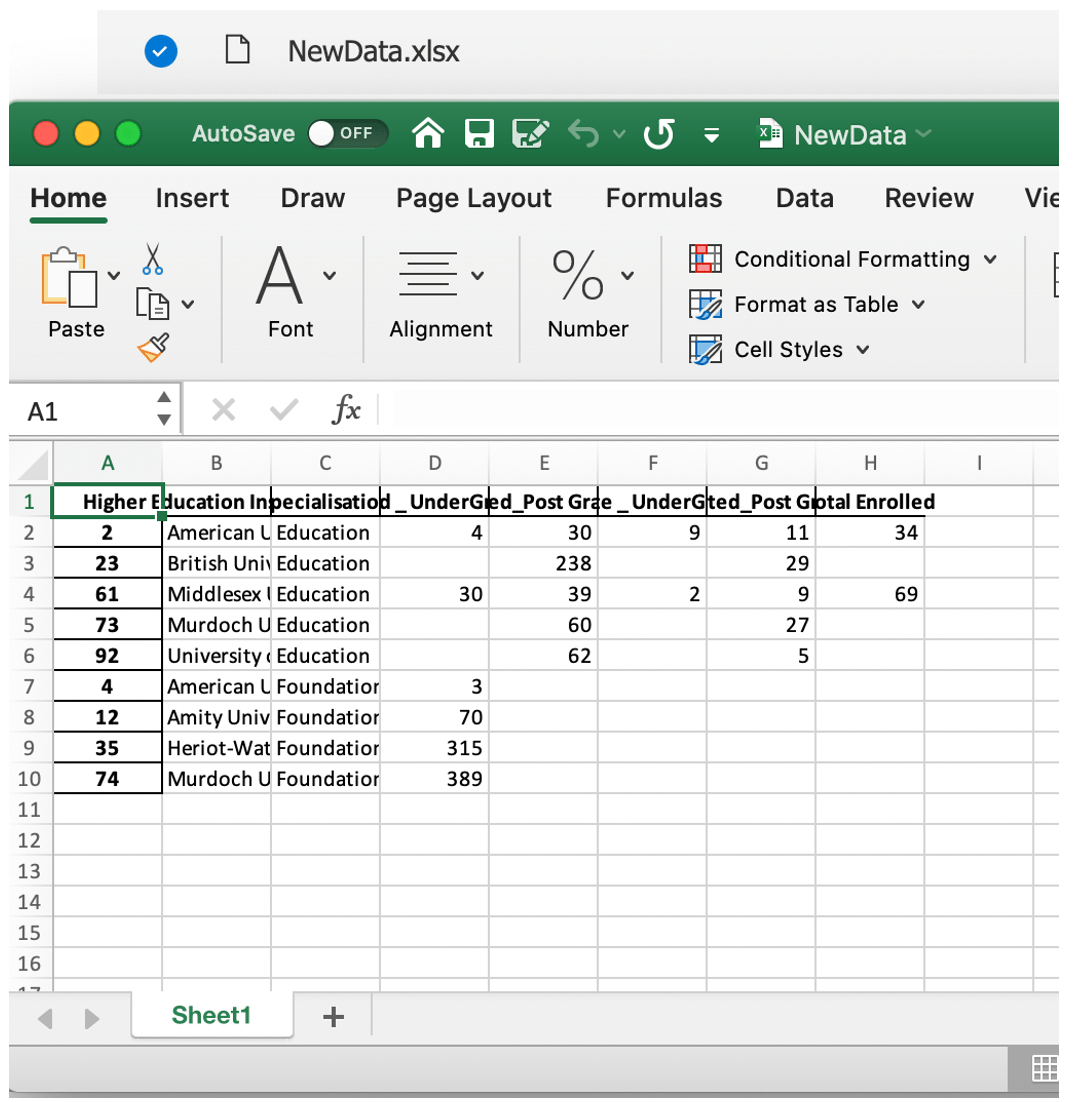## Summary of Pandas Commands